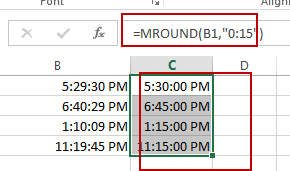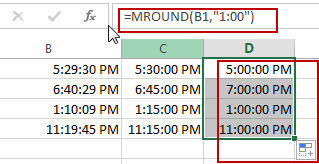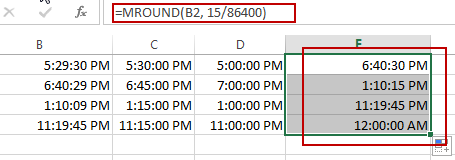## How to Round Time to Nearest Hour or Minute or Second in Excel

This post will guide you how to round time to nearest hour with a formula in Excel. How do I round off time values using the MROUND function in Excel. How to round time to the nearest minute or quarter hour in Excel.

## Round Time Values to Nearest 15 minutes

Assuming that you have a list of data in range B1:B4, in which contain time values. And if you want to round those time values to the nearest 15 minute interval, you can use a formula based on the MROUND function. Like this:

`=MROUND(B1,"0:15")`

Or

`=MROUND(B1,15/60/24)`

Type this formula into a cell C1, and press Enter key. Then drag the AutoFill Handle over to other cells.Note: the MROUND function will round to nearest values based on a supplied multiple.

## Round Time Values to Nearest 1 Hour

If you want to round time values to the nearest 1 hour, you can use the following formula based on the MROUND function. Like this:

`=MROUND(B1,"1:00")`

Type this formula into a cell D1, and press Enter key. Then drag the AutoFill Handle over to other cells.## Round Time Values to Nearest 15 seconds

If you want to round time values to the nearest 15 seconds, you can use the following formula based on the MROUND function. Like this:

`=MROUND(B2, 15/86400)`

Type this formula into a cell E1, and press Enter key. Then drag the AutoFill Handle over to other cells.